Test: Profit & Loss- 5

# Test: Profit & Loss- 5

Test Description

## 15 Questions MCQ Test UPSC Prelims Paper 2 CSAT - Quant, Verbal & Decision Making | Test: Profit & Loss- 5

Test: Profit & Loss- 5 for Quant 2022 is part of UPSC Prelims Paper 2 CSAT - Quant, Verbal & Decision Making preparation. The Test: Profit & Loss- 5 questions and answers have been prepared according to the Quant exam syllabus.The Test: Profit & Loss- 5 MCQs are made for Quant 2022 Exam. Find important definitions, questions, notes, meanings, examples, exercises, MCQs and online tests for Test: Profit & Loss- 5 below.
Solutions of Test: Profit & Loss- 5 questions in English are available as part of our UPSC Prelims Paper 2 CSAT - Quant, Verbal & Decision Making for Quant & Test: Profit & Loss- 5 solutions in Hindi for UPSC Prelims Paper 2 CSAT - Quant, Verbal & Decision Making course. Download more important topics, notes, lectures and mock test series for Quant Exam by signing up for free. Attempt Test: Profit & Loss- 5 | 15 questions in 30 minutes | Mock test for Quant preparation | Free important questions MCQ to study UPSC Prelims Paper 2 CSAT - Quant, Verbal & Decision Making for Quant Exam | Download free PDF with solutions
 1 Crore+ students have signed up on EduRev. Have you?
Test: Profit & Loss- 5 - Question 1

### A group of students prepared stuffed toys as part of their group activity. They spent Rs 100 on velvet, Rs 5 on thread and needle and Rs 27 on miscellaneous items. They made 30 toys, 50% of which were purchased by force by some senior students causing them a loss of 50%. At what % profit should they sell the remaining toys so as to gain 50% on their total cost?

Detailed Solution for Test: Profit & Loss- 5 - Question 1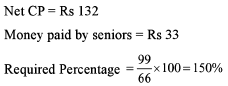Test: Profit & Loss- 5 - Question 2

### Read the passage below and solve the questions based on it, Patna-mart, sister concern of Wal-marL is planning to establish a mechanism to give discount, it is actually a Kind Ja Simsim kind of game where if you win any round, you will gel some discount specific to dial round, and it you lose, you will be losing al! the discounts so far won. Now, round l is hav ing discount coupons of 2%. Round 2 is having discount coupons of 4%. Round 3 is having discount coupons of 6%, And so on............... There are infinite round sand you can participate in all the rounds but you can participate in any round only once and before participating in round N (any particular round), you should have participated in round 1 - round N -1 (all die rounds before that particular round.) All the discounts won will be successive, eg., if you win Round 1 and then round 2 also, you will get a successive discount of 2% and then 4%. But again as the rule goes on. if you lose round 3, you will be losing ail the discount coupons won so far Bui despite losing round 3, you can participate in round 4 and hence. Q. What is the minimum number of rounds to obtain maximum possible discount?

Detailed Solution for Test: Profit & Loss- 5 - Question 2

Maximum possible discount in any case can be only 100% and any discount more than 100% is actually 100% only. Now, whatever is the discount, till the moment we get a discount of 100%, value of the article cannot be zero (even infinite discounts of 99.99% won’t make it equal to a discount of 100%) So, number of rounds needed to obtain 100% discount = 50.

Test: Profit & Loss- 5 - Question 3

### Read the passage below and solve the questions based on it, Patna-mart, sister concern of Wal-marL is planning to establish a mechanism to give discount, it is actually a Kind Ja Simsim kind of game where if you win any round, you will gel some discount specific to dial round, and it you lose, you will be losing al! the discounts so far won. Now, round l is hav ing discount coupons of 2%. Round 2 is having discount coupons of 4%. Round 3 is having discount coupons of 6%, And so on............... There are infinite round sand you can participate in all the rounds but you can participate in any round only once and before participating in round N (any particular round), you should have participated in round 1 - round N -1 (all die rounds before that particular round.) All the discounts won will be successive, eg., if you win Round 1 and then round 2 also, you will get a successive discount of 2% and then 4%. But again as the rule goes on. if you lose round 3, you will be losing ail the discount coupons won so far Bui despite losing round 3, you can participate in round 4 and hence. Q. What is the minimum possible discount after 20 rounds provided that customer has won a minimum of 18 rounds?

Detailed Solution for Test: Profit & Loss- 5 - Question 3

Despite the fact that a customer has won 18 rounds out of the first 20 rounds, if he looses in the 20th round, net discount = 0%

Test: Profit & Loss- 5 - Question 4

Had the CP been 10% less and SP been 10% more, profit % would have been double than that of earlier case

Q.

What is the profit % in original case?

Detailed Solution for Test: Profit & Loss- 5 - Question 4

Profit percentage as calculated above = 28.56%.

Test: Profit & Loss- 5 - Question 5

A shopkeeper marks up his goods by 40% and gives a discount of 10%. Apart from this, he uses a faulty balance also, which reads 1000 gm for 800 gm. What is his net profit percentage?

Detailed Solution for Test: Profit & Loss- 5 - Question 5

Since he sells the goods at a discount the selling price will be (40% - 10%) = 30% profit.

The profit is 30%.

Lets take the buying price as x.

The profit on the 1000g rather 1 kg will be 30% of x.

This gives us 0.3x

In reality this is the profit for 800 g.

The profit he gets for 1000g will be :

1000/800 × 0.3x = 0.375x

To change this to percentage it becomes :

0.375x/x × 100% = 37.5

The overall percentage profit gained by the seller will be given as : 37.5%

Test: Profit & Loss- 5 - Question 6

Read the passage below and solve the questions based on it, A shop has the following discount layers 1st layer - Buy Jn get 2 free. 2nd layer - Buy 2, get 3 free. 3rd layer - Buy 3, gel 4 free And so on there are infinite layers on the same pattern

Q.

For how many of these discount layers discount percentage offered is more than 50%?

Detailed Solution for Test: Profit & Loss- 5 - Question 6

Since in all these cases, more articles are given free than the purchased article, so the net discount is more than 50%. Hence in all these layers, the discount offered is more than 50%. (If the articles given free is equal to the articles purchased, discount percentage will be equal to 50%).

Test: Profit & Loss- 5 - Question 7

A bookseller marks his books at an advance of 69% on the actual cost of production. He allows a discount of 15% and also given a copy free for every dozen sold at a time. What rate per cent profit does the bookseller make, if books are sold in lots of 12?

Detailed Solution for Test: Profit & Loss- 5 - Question 7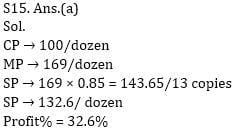Test: Profit & Loss- 5 - Question 8

Apublisher printed 3,000 copies of ‘Future Shock ’ at a cost of Rs 2,400. He gave 500 copies free to different philanthropic institutions. He allowed a discount of 25% on the published price and gave one copy free for every 25 copies bought at a time. He was able to sell all the copies in this manner. If the published price is Rs 3.25, then what is his overall gain or loss percentage in the whole transaction?

Detailed Solution for Test: Profit & Loss- 5 - Question 8

Cost Rs 2400

Published Price Rs 3.25

SP = 75/100 x 3.25 = Rs 2.4375

No. of free copy = (3000/25) = 120 + 500 = 620

So, total SP = 2380 x Rs 2.4375 = Rs 5801.25

Hence percentage gain = 5801.25 - 2400/2400 - 100 = 144%

Test: Profit & Loss- 5 - Question 9

A shopkeeper uses a “point of sales” software which prepares the bill for customers based on the number of units per unit. Both of the above are 2-digit numbers. While entering the data, he erroneously swaps the digits of both quantity of units and price. As a result, the closing stock balance is lowered by 72 units, and the sales value shown is Rs 1,368. How many units have actually been sold?

Detailed Solution for Test: Profit & Loss- 5 - Question 9

9(x - y ) = 72, x - y = 8

Therefore, only possibility is 19 or 91.

Test: Profit & Loss- 5 - Question 10

Mohit goes to furniture shop to buy a sofa set and a center table. He bargains for a 10% discount on the center table and 25% discount on sofa set. However, the shopkeeper, by mistake, interchanged the discount percentage figures while making the bill and mohit paid accordingly. When compared to what he should pay for his purchases, what percentage did mohit pay extra given that the center table costs 40% as much as the sofa set.

Detailed Solution for Test: Profit & Loss- 5 - Question 10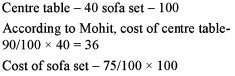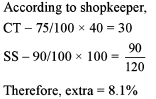Test: Profit & Loss- 5 - Question 11

A shopkeeper purchases a packet of 50 pens at Rs 10 per pen. He sells a part of the packet at a profit of 30%. On the remaining part, he incurs a loss of 10%. If his overall profit on the whole packet is 10%, the number of pens he sold at profit is

Detailed Solution for Test: Profit & Loss- 5 - Question 11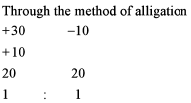Test: Profit & Loss- 5 - Question 12

Read the passage below and solve the questions based on it Newspaper major The Asian Times is dying to enter the city of Paperabad. where already two newspapers The Deccan
City and Good Morning Pape rah ad are in circulation. The population of the city is 35 lakhs of which, according to the recent survey, 60% read newspaper. The two evening newspapers sell at Rs 1.75 per copy on weekdays and Rs3.00 per copy on Sundays. The Asian Times promised its readers newspapers at Rs 1.50 per copy per day. Since people w'crc aware of the quality7 of the paper, the management thought that it can capture a major chunk of the newspaper reading population. On weekdays, the operational cost of the The Asian Times w-crc Rs 62.500 per day and the cost for the material and printing charges was Rs 2.75 per newspaper. For the Sunday edition the cost of the newspaper per copy was Rs 3.00 and the operational cost were Rs 75,000. The number of the copies of tire newspaper The Asian Tunes sold on Sunday was 16.66% less than the number of copies sold on any of the week days. The number of copies sold on any weekday being the same. The amount collected through the advertisement on any weekday was the same and equaled Rs 2.50,000 per day while for Sunday it was 4.35.000.

Q.

If 50,000 of the readers of The Deccan City now read The Asian Times on all weekdays, how much profit would The Asian Times expect to make every week?

Detailed Solution for Test: Profit & Loss- 5 - Question 12

Since we are not having any information regarding the total number of copies sold (the information is talking about the reader of “The Deccan City”), we cannot determine the profit.

Test: Profit & Loss- 5 - Question 13

Read the passage below and solve the questions based on it.
In the ' Gel-One'' supermarket, a new software was installed in the computerized billing section which made the calculations of each of the item sold very easy on the first day of the operations. To verify the workability of the software only one of the item was billed Oil the computer.
Due to some technical problem in the software, it reversed both the numbers of the item sold and price per piece as a result of which the closing of the slock items showed 63 items less and the value on the items still left was show n Rs 936.
It was known that both die slocks left and die price per piece are two digit numbers.

Q.

What is the correct collection for the day if 44 pieces were sold?

Detailed Solution for Test: Profit & Loss- 5 - Question 13

Rate = 936/18= 52

It is given that price/piece was also reversed.

So, price/piece = Rs 25

Collection = Rs 25 x 44 = Rs 1100

Test: Profit & Loss- 5 - Question 14

Read the passage below and solve the questions based on it.
The following table shows the relationship between production volumes, unit price and unit cost of a particular product. Refer lo the following table to answer the questions that Follow: Contribution == volume x (price - cost)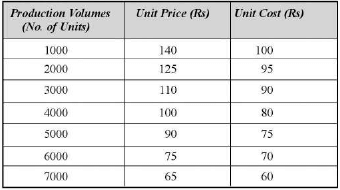Q.

At what volume will the contribution be maximum?

Detailed Solution for Test: Profit & Loss- 5 - Question 14

Using the options,

(a) 2,000 units, C = 2,000 x 30 = Rs 60,000

(b) 3,000 units, C = 3,000 x 20 = Rs 60,000

(c) 4,000 units, C = 4,000 x 20 = Rs 80,000

(d) 5,000 units, C = 5,000 x 15 = Rs 75,000

Test: Profit & Loss- 5 - Question 15

Read the passage below and solve the questions based on it.
The following table shows the relationship between production volumes, unit price and unit cost of a particular product. Refer lo the following table to answer the questions that Follow: Contribution == volume x (price - cost)Q.

A breakeven point is the volume of production at which for the first time the contribution equals fixed costs. If the fixed costs are Rs 60,000, then the production level at which the break even is reached, is

Detailed Solution for Test: Profit & Loss- 5 - Question 15

From question number 38 At 2,000 units, C = Rs 60,000.

## UPSC Prelims Paper 2 CSAT - Quant, Verbal & Decision Making

67 videos|50 docs|151 tests
 Use Code STAYHOME200 and get INR 200 additional OFF Use Coupon Code
Information about Test: Profit & Loss- 5 Page
In this test you can find the Exam questions for Test: Profit & Loss- 5 solved & explained in the simplest way possible. Besides giving Questions and answers for Test: Profit & Loss- 5, EduRev gives you an ample number of Online tests for practice

## UPSC Prelims Paper 2 CSAT - Quant, Verbal & Decision Making

67 videos|50 docs|151 tests